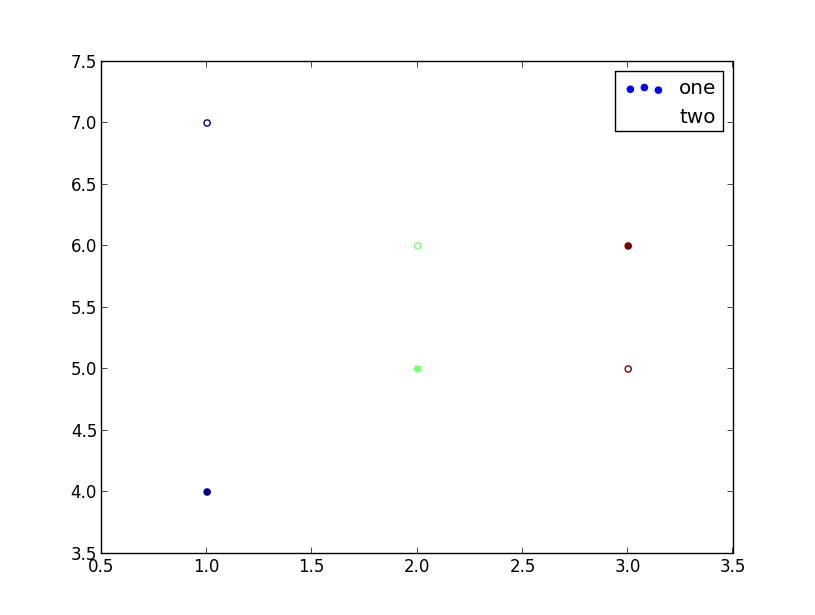# open circles aren't showing up in the legend box

Using matplotlib.pyplot I create a scatter plot using two symbols, an open circle, and a filled circle. The legend for this plot shows both labels, but there is no symbol for the open circles (see missing_legend_circles.png). Switching to matplotlib.pylab yields the same result. However, from an “ipython --pylab” session the open circles are displayed in the legend. This has me more baffled than usual. Here’s the code to reproduce the enclosed figure:

import matplotlib.pyplot as plt

fig = plt.figure()

plot.scatter([1, 2, 3], [4, 5, 6], c = [0.2, 0.4, 0.6], label = ‘one’,

``````     cmap = 'jet', marker = 'o', edgecolor = 'face')
``````

plot.scatter([1, 2, 3], [7, 6, 5], c = [0.2, 0.4, 0.6], label = ‘two’,

cmap = ‘jet’, marker = ‘o’, edgecolor = ‘face’,

``````      facecolor = 'none')
``````

plot.legend()

plt.show()

This is with matplotlib 1.1 and 1.2 on Windows 7 with the TkAgg, WxAgg and WX backends.

Thank you for any help.

– Joe LouderbackI'm not quite sure what /should/ happen when you set the `facecolor` to
'none' and the `edgecolor` to 'face'. I'm surprised anything shows up at
the axes at all. But if you remove the `edgecolor` kwarg things look better:

import matplotlib.pyplot as plt
fig = plt.figure()
plot.scatter([1, 2, 3], [4, 5, 6], c = [0.2, 0.4, 0.6], label = 'one',
cmap = 'jet', marker = 'o', edgecolor = 'face')

plot.scatter([1, 2, 3], [7, 6, 5], c = [0.2, 0.4, 0.6], label = 'two',
cmap = 'jet', marker = 'o', facecolor='none')
plot.legend()
plt.show()

···

On Wed, Jan 2, 2013 at 4:46 PM, Joe Louderback <jglouderback@...287...>wrote:

import matplotlib.pyplot as plt

fig = plt.figure()

plot.scatter([1, 2, 3], [4, 5, 6], c = [0.2, 0.4, 0.6], label = 'one',
cmap = 'jet', marker = 'o', edgecolor = 'face')

plot.scatter([1, 2, 3], [7, 6, 5], c = [0.2, 0.4, 0.6], label = 'two',
cmap = 'jet', marker = 'o', edgecolor = 'face',
facecolor = 'none')
plot.legend()
plt.show()

I doubt there is a color called 'face', according to documentation:

In : setp(mat.collections.PathCollection,'edgecolor')
edgecolor: matplotlib color arg or sequence of rgba tuples

probably because matplotlib doesn't know how rend the color 'face'?

Chao

···

--
View this message in context: http://matplotlib.1069221.n5.nabble.com/open-circles-aren-t-showing-up-in-the-legend-box-tp40136p40138.html
Sent from the matplotlib - users mailing list archive at Nabble.com.

Joe,

I think the problem is the edgecolor='face' in the scatter call for the
open circles. For me when I omit that, it all works. I'd also note
that calling legend after draw results in the legend showing the circles
correctly colored.

Regards,
Jon

···

On Wed, 2013-01-02 at 16:46 -0800, Joe Louderback wrote:

Using matplotlib.pyplot I create a scatter plot using two symbols, an
open circle, and a filled circle. The legend for this plot shows both
labels, but there is no symbol for the open circles (see
missing_legend_circles.png). Switching to matplotlib.pylab yields the
same result. However, from an "ipython --pylab" session the open
circles are displayed in the legend. This has me more baffled than
usual. Here's the code to reproduce the enclosed figure:

import matplotlib.pyplot as plt

fig = plt.figure()

plot.scatter([1, 2, 3], [4, 5, 6], c = [0.2, 0.4, 0.6], label =
'one',
cmap = 'jet', marker = 'o', edgecolor = 'face')

plot.scatter([1, 2, 3], [7, 6, 5], c = [0.2, 0.4, 0.6], label = 'two',
cmap = 'jet', marker = 'o', edgecolor = 'face',
facecolor = 'none')
plot.legend()
plt.show()

This is with matplotlib 1.1 and 1.2 on Windows 7 with the TkAgg, WxAgg
and WX backends.

Thank you for any help.

-- Joe Louderback

--
______________________________________________________________
Jonathan D. Slavin Harvard-Smithsonian CfA
jslavin@...1081... 60 Garden Street, MS 83
phone: (617) 496-7981 Cambridge, MA 02138-1516
cell: (781) 363-0035 USA
______________________________________________________________

Thank you everyone for your answers. My legend is no longer defaced since
I de-faced the call to scatter()